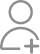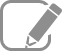Take Class 12 Tuition from the Best Tutors

•Affordable fees
•1-1 or Group class
•Flexible Timings
•Verified Tutors

Search in

# What is the difference between position vector and displacement vector?Follow 29AnswerIIT grad Tutor who can teach all subjects

Change in position vector is displacement.

Mathematics and Science Tutor Having 12 yrs of Experience.

Position vector as the vector starting from origin to the point but displacement vector as the vector joining initial and final position.

Home Tutor from 4 year. And journey is ahead

The position vector is a coordinate of an object in a plane or space concerning origin and direction is directed from the source toward the coordinate. Whereas Displacement vector is the shortest distance between the initial and final point of a whole continuous motion, and direction is from starting...

The position vector is a coordinate of an object in a plane or space concerning origin and direction is directed from the source toward the coordinate. Whereas Displacement vector is the shortest distance between the initial and final point of a whole continuous motion, and direction is from starting point to endpoint.

The displacement vector is the difference between the two-position vector.

Positon vector is used to locate an object in space or a plane.

Physics expert with 7 years of experience taught in airforce and kv schools

The position vector is the distance between the starting point to the position of the object while the displacement vector is the length between the initial point to the final point. The displacement vector is always a position vector, but the position vector doesn't need always to be a displacement...

The position vector is the distance between the starting point to the position of the object while the displacement vector is the length between the initial point to the final point.

The displacement vector is always a position vector, but the position vector doesn't need always to be a displacement vector; it may be or may not be according to the conditions.

Position Vector gives the position, i.e., location or coordinate of the particle with respect to reference point called origin along with its direction with any reference axis such as X-axis. In contrast, displacement vector gives the shortest distance of the particle from its' start point to endpoint...

Position Vector gives the position, i.e., location or coordinate of the particle with respect to reference point called origin along with its direction with any reference axis such as X-axis. In contrast, displacement vector gives the shortest distance of the particle from its' start point to endpoint along with its direction with any of the reference axis say X-axis or with any fixed direction say North.

The position vector of a particle is defined as the vector starting from the origin to the point where the particle is. The displacement vector of a particle is defined as the vector joining its initial position to its final position. In other words, the displacement vector is a change in position ve...

The position vector of a particle is defined as the vector starting from the origin to the point where the particle is. The displacement vector of a particle is defined as the vector joining its initial position to its final position. In other words, the displacement vector is a change in position vector.

Yes I'm three years experience teacher and provide best results

The position vector tells where an object is relative to a “fixed” origin. The displacement vector indicates where an object is relative to where it was in its last position.

Physics Teacher (PhD) with more than 10 years of experience in scientific field

The position vector is a vector that extends from a reference point (usually taken as the origin of the coordinate system) to the object whose location has to be determined. As the object moves, its position changes with respect to time. The displacement vector is the difference in the final position...

The position vector is a vector that extends from a reference point (usually taken as the origin of the coordinate system) to the object whose location has to be determined. As the object moves, its position changes with respect to time. The displacement vector is the difference in the final position vector and initial position vector.

Position vectors are point vectors which merely shows the exact position of a particle/body in any plane. Displacement vectors are the change in position vectors (final-initial).

15+ Years of Experience Teaching Physics and Maths in CBSE Schools

Position vector describes the position of any point in space. It is a vector drawn from the origin towards that point on space. The displacement vector is a vector that gives information about the change in position of the object in motion in a particular interval of time.

Related Questions23/10/2018
527/05/2020
143Now ask question in any of the 1000+ Categories, and get Answers from Tutors and Trainers on UrbanPro.com

Related Lessons

Introduction to physics
Hello everyone, In these lesson we are learn about introduction of phyics. I give you first of all. a Example if we want to play any game we should know rules of that game that's like if...
S

Newton's Laws
Newton's Laws -- 1st Law -- the body is in rest until the experience any type of external force. I.e F = 0. Consider a following. Example. Newton's 2nd law-- when we apply any type of force...
S

Frame of Reference
Frame of reference:When the position of a particle in space changes with time, it is said to be in motion. To describe motion, a frame of reference is needed first and foremost. The set of spatial coordinates...How to Solve Numericals in Physics?
Are you scared of numerical? Do you think you don't understand them? If yes, then read ahead. See, it might be any exam, but numerical won't leave your back. So let's talk about common problems and their...Understanding Conservative and Non Conservative Forces
While teaching my students, I found them to fumble in this topic, so I am writing this lesson to tell you all in simple terms what the topic is:Consider two cases in both cases; a block moves from A to...Find Class 12 Tuition near you

Looking for Class 12 Tuition ?

Learn from the Best Tutors on UrbanPro

Are you a Tutor or Training Institute?

Join UrbanPro Today to find students near you
X

### Looking for Class 12 Tuition Classes?

The best tutors for Class 12 Tuition Classes are on UrbanPro

• Select the best Tutor
• Book & Attend a Free Demo
• Pay and start Learning### Take Class 12 Tuition with the Best Tutors

The best Tutors for Class 12 Tuition Classes are on UrbanPro